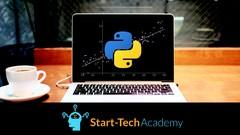# Complete Linear Regression Analysis in Python [100% OFF UDEMY COUPON]COURSE AUTHOR –

## What you'll learn :

1. Learn how to solve real life problem using the Linear Regression technique
2. Preliminary analysis of data using Univariate and Bivariate analysis before running Linear regression
3. Predict future outcomes basis past data by implementing Simplest Machine Learning algorithm
4. Understand how to interpret the result of Linear Regression model and translate them into actionable insight
5. Understanding of basics of statistics and concepts of Machine Learning
6. Indepth knowledge of data collection and data preprocessing for Machine Learning Linear Regression problem
7. Learn advanced variations of OLS method of Linear Regression
8. Course contains a end-to-end DIY project to implement your learnings from the lectures
9. How to convert business problem into a Machine learning Linear Regression problem
10. Basic statistics using Numpy library in Python
11. Data representation using Seaborn library in Python
12. Linear Regression technique of Machine Learning using Scikit Learn and Statsmodel libraries of Python

## Apply this Coupon :

CFWELQ

If Coupon is Expired/Invalid !!!

Possible Reasons!

• One coupon can last maximum for 2 days. Your are Late
• Author revoked the coupon. Author have rights to revoke the coupon any time.You missed it Bad Luck!!!

But Don’t worry , if you missed it, join our groups to receive all coupon instantly whenever it is posted.